# Errorbar graph in Python using Matplotlib

Error bars function used as graphical enhancement that visualizes the variability of the plotted data on a Cartesian graph. Error bars can be applied to graphs to provide an additional layer of detail on the presented data. As you can see in below graphs.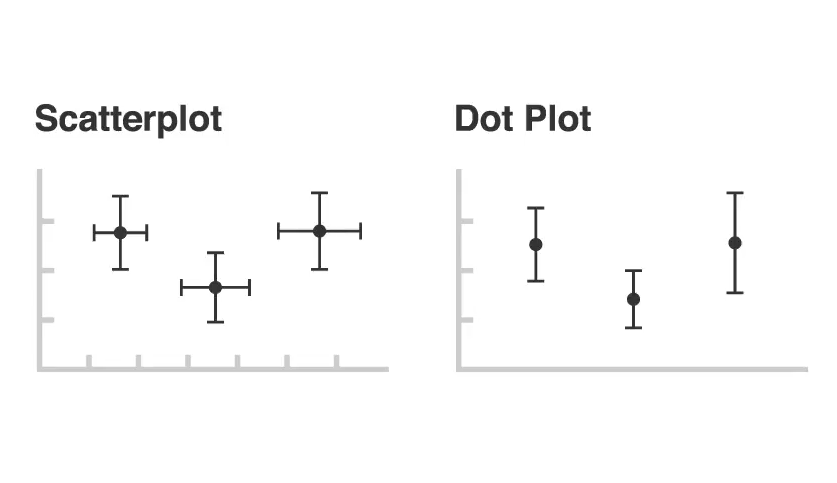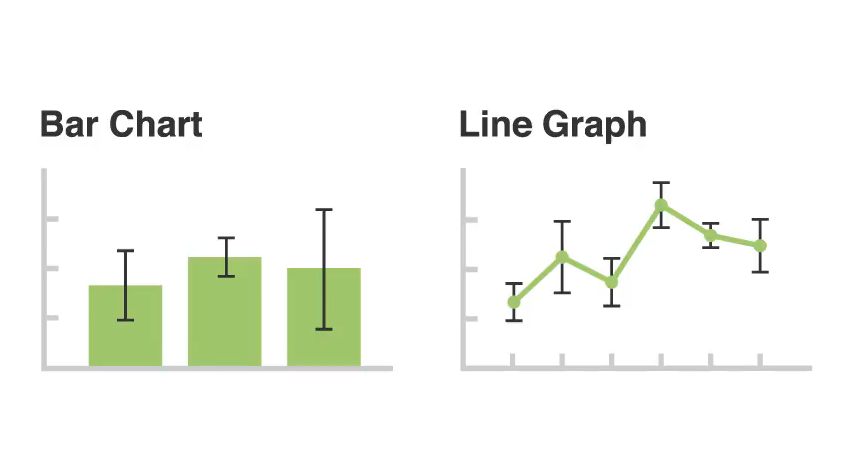Error bars help you indicate estimated error or uncertainty to give a general sense of how precise a measurement is this is done through the use of markers drawn over the original graph and its data points. To visualize this information error bars work by drawing lines that extend from the center of the plotted data point or edge with bar charts the length of an error bar helps to reveal uncertainty of a data point as shown in the below graph. A short error bar shows that values are concentrated signaling that the plotted averaged value is more likely while a long error bar would indicate that the values are more spread out and less reliable. also depending on the type of data. the length of each pair of error bars tends to be of equal length on both sides, however, if the data is skewed then the lengths on each side would be unbalanced.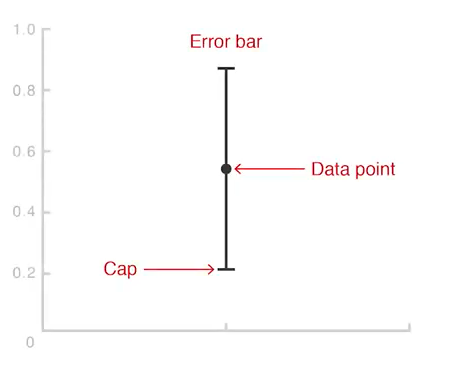Error bars always run parallel to a quantity of scale axis so they can be displayed either vertically or horizontally depending on whether the quantitative scale is on the y-axis or x-axis if there are two quantity of scales and two pairs of arrow bars can be used for both axes.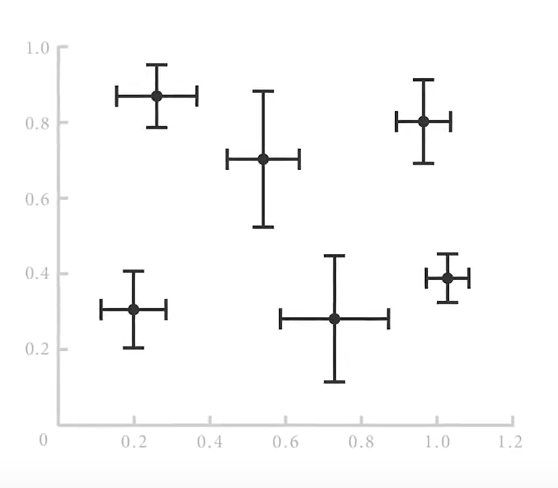Let see an example of errorbar how it works.

Creating a Simple Graph.

 `# importing matplotlib  ` `import` `matplotlib.pyplot as plt ` ` `  `# making a simple plot ` `x ``=``[``1``, ``2``, ``3``, ``4``, ``5``, ``6``, ``7``] ` `y ``=``[``1``, ``2``, ``1``, ``2``, ``1``, ``2``, ``1``] ` ` `  `# ploting graph ` `plt.plot(x, y) `

Output: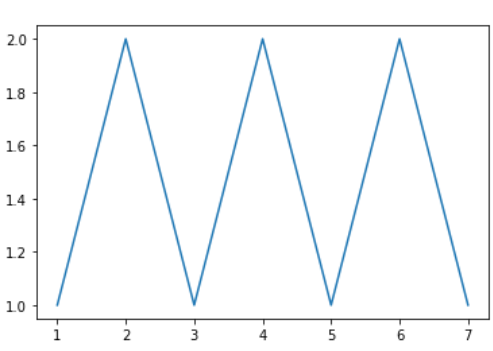Example 1: Adding Some error in y value.

 `# importing matplotlib ` `import` `matplotlib.pyplot as plt  ` ` `  ` `  `# making a simple plot ` `x ``=``[``1``, ``2``, ``3``, ``4``, ``5``, ``6``, ``7``] ` `y ``=``[``1``, ``2``, ``1``, ``2``, ``1``, ``2``, ``1``] ` ` `  `# creating error ` `y_error ``=` `0.2` ` `  `# ploting graph ` `plt.plot(x, y) ` ` `  `plt.errorbar(x, y, ` `             ``yerr ``=` `y_error, ` `             ``fmt ``=``'o'``) `

Output: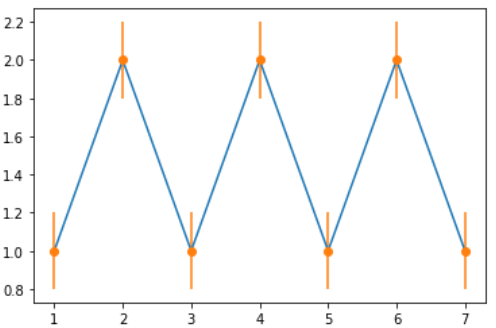Example 2: Adding Some error in x value.

 `# importing matplotlib ` `import` `matplotlib.pyplot as plt  ` ` `  `# making a simple plot ` `x ``=``[``1``, ``2``, ``3``, ``4``, ``5``, ``6``, ``7``] ` `y ``=``[``1``, ``2``, ``1``, ``2``, ``1``, ``2``, ``1``] ` ` `  `# creating error ` `x_error ``=` `0.5` ` `  `# ploting graph ` `plt.plot(x, y) ` `plt.errorbar(x, y, ` `             ``xerr ``=` `x_error, ` `             ``fmt ``=``'o'``) `

Output: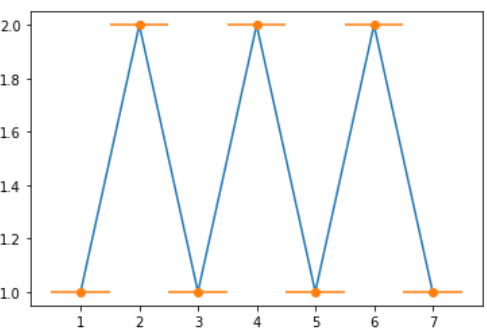Example 3: Adding error in x & y

 `# importing matplotlib ` `import` `matplotlib.pyplot as plt  ` ` `  ` `  `# making a simple plot ` `x ``=``[``1``, ``2``, ``3``, ``4``, ``5``, ``6``, ``7``] ` `y ``=``[``1``, ``2``, ``1``, ``2``, ``1``, ``2``, ``1``] ` ` `  `# creating error ` `x_error ``=` `0.5` `y_error ``=` `0.3` ` `  `# ploting graph ` `plt.plot(x, y) ` `plt.errorbar(x, y,  ` `             ``yerr ``=` `y_error,  ` `             ``xerr ``=` `x_error,  ` `             ``fmt ``=``'o'``) `

Output: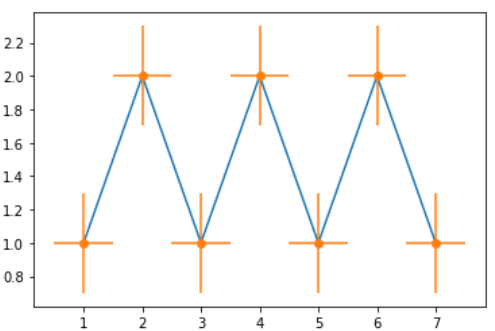Example 4: Adding variable error in x and y

 `# importing matplotlib  ` `import` `matplotlib.pyplot as plt ` ` `  ` `  `# making a simple plot ` `x ``=``[``1``, ``2``, ``3``, ``4``, ``5``] ` `y ``=``[``1``, ``2``, ``1``, ``2``, ``1``] ` ` `  `# creating error ` `y_errormin ``=``[``0.1``, ``0.5``, ``0.9``, ` `             ``0.1``, ``0.9``] ` `y_errormax ``=``[``0.2``, ``0.4``, ``0.6``,  ` `             ``0.4``, ``0.2``] ` ` `  `x_error ``=` `0.5` `y_error ``=``[y_errormin, y_errormax] ` ` `  `# ploting graph ` `# plt.plot(x, y) ` `plt.errorbar(x, y, ` `             ``yerr ``=` `y_error, ` `             ``xerr ``=` `x_error,  ` `             ``fmt ``=``'o'``) `

Output: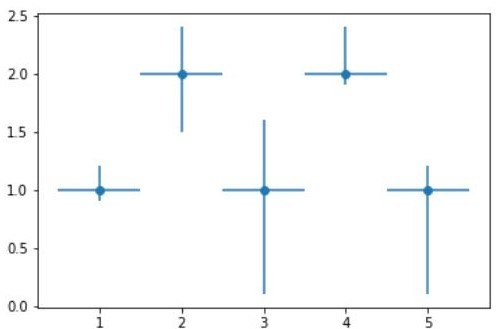Example 5:

 `# import require modules  ` `import` `numpy as np ` `import` `matplotlib.pyplot as plt ` ` `  ` `  `# defining our function  ` `x ``=` `np.arange(``10``)``/``10`  `y ``=` `(x ``+` `0.1``)``*``*``2` ` `  `# defing our error  ` `y_error ``=` `np.linspace(``0.05``, ``0.2``, ``10``) ` ` `  `# ploting our function and  ` `# error bar ` `plt.plot(x, y) ` ` `  `plt.errorbar(x, y, yerr ``=` `y_error, fmt ``=``'o'``) `

Output: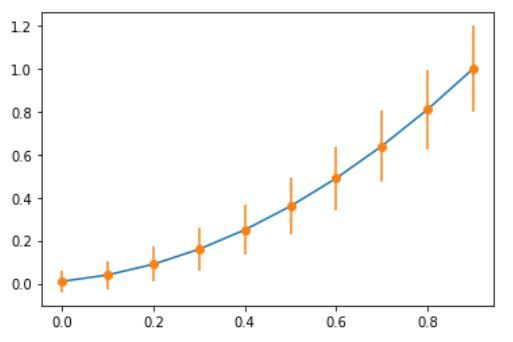Attention geek! Strengthen your foundations with the Python Programming Foundation Course and learn the basics.

To begin with, your interview preparations Enhance your Data Structures concepts with the Python DS Course.

My Personal Notes arrow_drop_upCheck out this Author's contributed articles.

If you like GeeksforGeeks and would like to contribute, you can also write an article using contribute.geeksforgeeks.org or mail your article to contribute@geeksforgeeks.org. See your article appearing on the GeeksforGeeks main page and help other Geeks.

Please Improve this article if you find anything incorrect by clicking on the "Improve Article" button below.